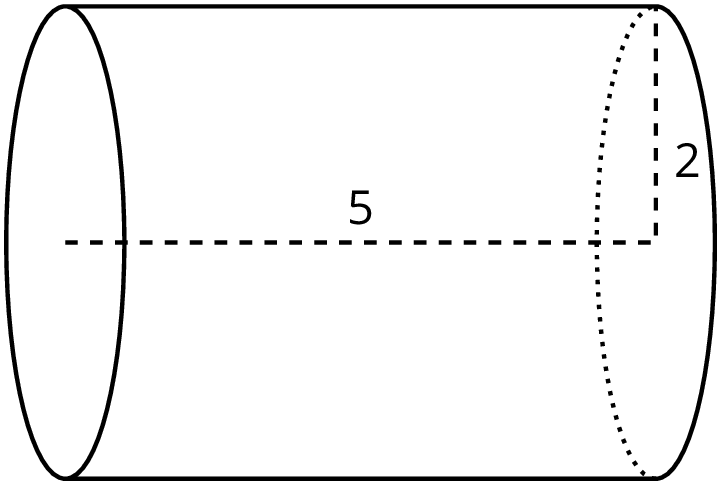## Inputs and Outputs

This week, your student will be working with functions. A function is a rule that produces a single output for a given input.

Not all rules are functions. For example, here’s a rule: the input is “first letter of the month” and the output is “the month.” If the input is J, what is the output? A function must give a single output, but in this case the output of this rule could be January, June, or July, so the rule is not a function.

Here is an example of a rule that is a function: input a number, square it, then multiply the result by $\pi$. Using $r$ for the input and $A$ for the output, we can draw a diagram to represent the function:We could also represent this function with an equation, $A=\pi r^2$. We say that the input of the function, $r$, is the independent variable and the output of the function, $A$, is the dependent variable. We can choose any value for $r$, and then the value of $A$ depends on the value of $r$. We could also represent this function with a table or as a graph. Depending on the question we investigate, different representations have different advantages. You may recognize this rule and know that the area of a circle depends on its radius.

Jada can buy peanuts for \$0.20 per ounce and raisins for \$0.25 per ounce. She has \$12 to spend on peanuts and raisins to make trail mix for her hiking group. 1. How much would 10 ounces of peanuts and 16 ounces of raisins cost? How much money would Jada have left? 2. Using$p$for pounds of peanuts and$r$for pounds of raisins, an equation relating how much of each they buy for a total of \$12 is $0.2p+0.25r=12$. If Jada wants 20 ounces of raisins, how many ounces of peanuts can she afford?
3. Jada knows she can rewrite the equation as $r=48-0.8p$. In Jada’s equation, which is the independent variable? Which is the dependent variable?

Solution:

1. 10 ounces of peanuts would cost \$2 since$0.2\boldcdot 10=2$. 16 ounces of raisins would cost \$4 since $0.25\boldcdot 16=4$. Together, they would cost Jada \$6, leaving her with \$6.
2. 35 ounces of peanuts. If Jada wants 20 ounces of raisins, then $0.2p+0.25 \boldcdot 20=12$ must be true, which means $p=35$.
3. $p$ is the independent variable and $r$ is the dependent variable for Jada’s equation.

## Linear Functions and Rates of Change

This week, your student will be working with graphs of functions. The graph of a function is all the pairs (input, output), plotted in the coordinate plane. By convention, we always put the input first, which means the inputs are represented on the horizontal axis and the outputs on the vertical axis.

For a graph representing a context, it is important to specify the quantities represented on each axis. For example this graph shows Elena’s distance as a function of time. If it is distance from home, then Elena starts at some distance from home (maybe at her friend’s house), moves further away from her home (maybe to a park), stays there a while, and then returns home. If it is distance from school, the story is different.The story also changes depending on the scale on the axes: is distance measured in miles and time in hours, or is distance measured in meters and time in seconds?

Match each of the following situations with a graph (you can use a graph multiple times). Define possible inputs and outputs, and label the axes.

1. Noah pours the same amount of milk from a bottle every morning.
2. A plant grows the same amount every week.
3. The day started very warm but then it got colder.
4. A cylindrical glass contains some partially melted ice. The more water you pour in, the higher the water level.Solution:

1. Graph B, input is time in days, output is amount of milk in the bottle
2. Graph A, input is time in weeks, output is height of plant
3. Graph C, input is time in hours, output is temperature
4. Graph A, input is volume of water, output is height of water

In each case, the horizontal axis is labeled with the input, and the vertical axis is labeled with the output.

## Cylinders and Cones

This week your student will be working with volumes of three-dimensional objects. We can determine the volume of a cylinder with radius $r$ and height $h$ using two ideas that we’ve seen before:

• The volume of a rectangular prism is a result of multiplying the area of its base by its height.
• The base of the cylinder is a circle with radius $r$, so the base area is $\pi r^2$.

Just like a rectangular prism, the volume of a cylinder is the area of the base times the height. For example, let’s say we have a cylinder whose radius is 2 cm and whose height is 5 cm like the one shown here: